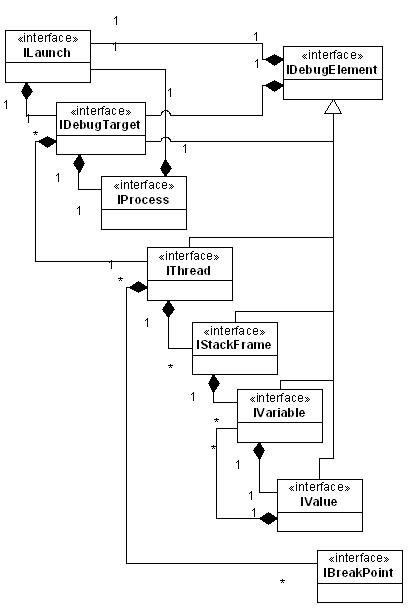## Friday, January 30, 2009

### Formal grammars, parsing etc. for dummies

I thought it may be useful to post summaries of what I have been reading recently. In this post, I will try to explain some basic grammar and parsing stuff in as simple way as I can (omitting all the academic complacency) .

A grammar defines a language with a set of rules of type x -> y, where x is left hand side of the rule and y is right hand side of the rule. x and y can be either expandable or literals depending on grammar type (math zealot? read terminals and non-terminals).

Chomsky hierarchy defines four levels of grammar (although these are not the only grammar types in literature but Chomsky is cool dude in linguistic literature, hence the list):

0. Unrestricted grammar:

Languages based on such grammar can have any rule for derivation (which usually means any text can belong to a possible unrestricted grammar like 'yo dawg', where 'yo dawg -> u'). However, it is possible to define a language with unrestricted grammar which is also Turing complete. A language based on unrestricted grammar is extremely difficult to parse, but not completely impossible. Such language doesn't necessarily make sense, e.g. It is possible to create a math expression such as this 1++1=1--*1=1 in an unrestricted language, as well as an unrestricted English statement such as this: "all your bases are belong to us".

1. Context sensitive grammar:

A language defined with CSG may represent all the natural languages. Such grammar is defined with a set of rules where both sides may have expandable symbols (e.g. "a" B "c" -> "a" "x" "c"). It is very difficult to build a parser which can parse CSG.

2. Context free grammar:

All programming language grammars are context free grammars. It can have only one expandable symbol on left hand side of a grammar rule and is very easy to parse. E.g. X -> "if" (y) z | "if" (y) z "else" (X), where y and z can be represented as other statements of the same grammar. There are several well known parsing techniques to parse CFGs such as:

- LL(1)-LL(k) with a recursive descent parser (top down parsers with look-aheads 1 to k) and,
- Bottom up parsers such as LR(1) and LALR which can parse more CFGs than LL parsers (generally shift reduce parsers).

LL parser starts with Left to right and always expands Left expandable symbol first (think of queue) where as LR starts with Left to right but expands Right symbol first (think of stack).

3. Regular grammar:

A regular expression is a language defined by a regular grammar. It is represented as X -> "t" | "t" Y, where Y represents expandable symbol and always follows literal (if it exists). E.g. ab*, where * may be an expandable symbol defined to mean one or more occurrence of the literal it followed as a grammar rule.

Each grammar type above is a subset of previous grammar type, meaning every regular grammar is context free grammar, every context free grammar is also context sensitive grammar and so on.

## Wednesday, January 21, 2009

### Mutual recursion fun with Eclipse Debug model

I rarely come across real-world data structures which are inherently mutually recursive (a.k.a. Daisy chain recursion in CS literature). Recently, while writing a plug-in related to eclipse.debug, I came across this interesting data structure.Source Eclipse.org

Observe the IVariable ←→ IValue relationship (although most relationships in this object-model is mutually recursive), given an implementation of this model (say JDI) how do you clone an object-tree representing variables on a stack-frame?

The answer is simple: assuming implementation classes Variable and Value just write a simple function to clone variables one by one:

`public class Variable {…private Value value;…public static Variable create(IVariable var) {  Variable v = new Variable() ;  try {      v.setName(var.getName());      v.setType(var.getReferenceTypeName());      v.setValue(Value.create(var.getValue()));      return v;  } catch (DebugException e) {      ..  }}}`
Similarly for Value class:

`public class Value {…private  Variable[] variables;…public static Value create(IValue var) {    Value v = new Value() ;    try {        v.setType(var.getReferenceTypeName());        v.setValue(...);        IVariable[] variables = value.getVariables();        if (variables != null) {            for (IVariable variable : variables)                v.add(Variable.create(variable));        }        return v;    } catch (DebugException e) {        ..    }}}`

Statement 'Variable.create(yourJDIVariable);' clones entire JDI object. This is one of those intuitive recursion examples which you die to find out IRL. Interesting, isn't it?

This is how your regular stack-frame looks (Variables view):And this is how it looks when you represent it using an example debug model :